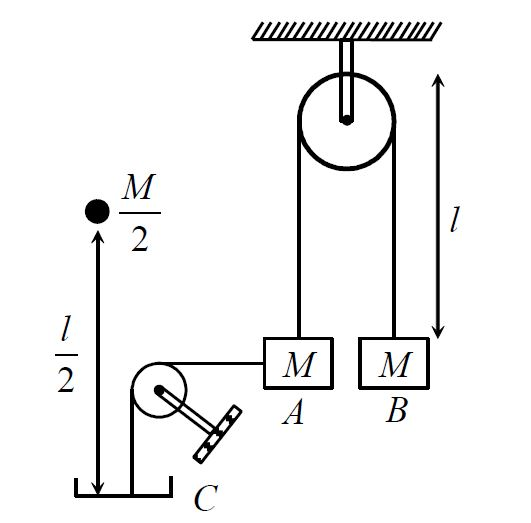# Pulleys+Impusle+Acceleartion!Two blocks $A$ and $B$ of same mass $M$ are connected with each other with an ideal string of length $2l$ passing over an ideal pulley. The block $A$ is connected to a light pan $C$ with an ideal string as shown in figure. A particle of mass $\frac{M}{2}$ is dropped on pan from height $\frac{l}{2}$ as shown. If collision between particle and pan is plastic, acceleration of $B$ just after the collision is?

×